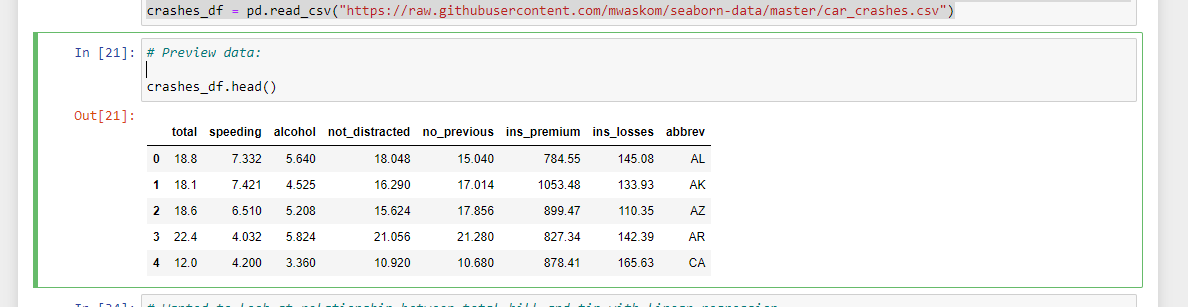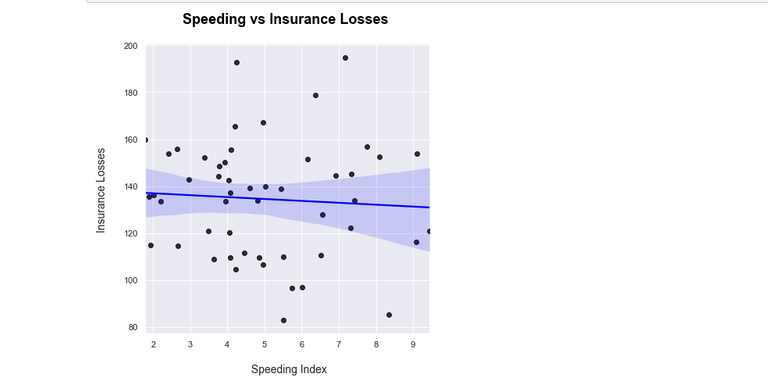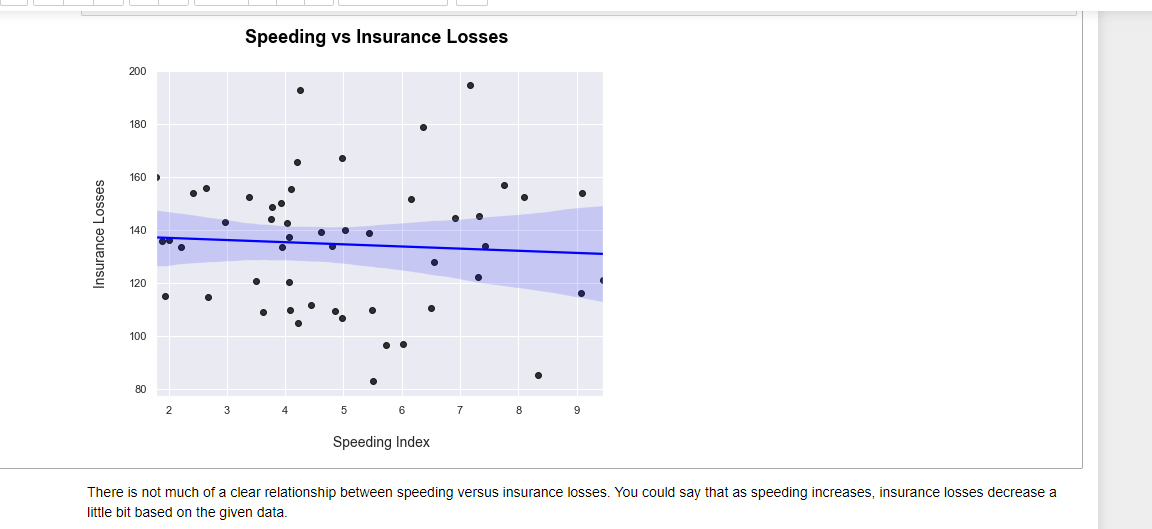# Regression Lines In Python & Seaborn

in STEMGeeks2 years ago

Hi there. In this post, I cover plotting regression lines or line of best fits to scatter plots in Python with matplotlib and seaborn.Pixabay Image Source

## Topics

• The Dataset
• Seaborn's regplot In Python
• lmPlot In Python's Seaborn

## The Dataset

The dataset that I use here is from the website https://github.com/mwaskom/seaborn-data. This dataset can also be obtained with the use of the seaborn library in Python. As I do prefer to load `.csv` files from the web I provide the link to this dataset in the code.

``````import pandas as pd
import matplotlib.pyplot as plt
import seaborn as sns
``````

The `.csv` dataset is loaded with the use of `.read_csv()` from pandas.

``````# Load data on car crashes:

``````

The data can be viewed with `crashes_df.head()`.## lmPlot In Python's Seaborn

One of the ways to display a scatter plot with a line of best fit is with the use seaborn's lmplot. The more technical phrase for a line of best fit is a regression line.

I want to see the relationship between speeding versus insurance losses in this dataset. With `sns.lmplot()`, you need to specify the columns being used from the given data. I set the scatter plot point colours to be black and the line colour to be blue. Labels & a title are added on with matplotlib.

``````# Wanted to look at relationship between total_bill and tip with linear regression.
# Lmplot method:

sns.lmplot(x = 'speeding', y = 'ins_losses', data = crashes_df,
height = 6,
scatter_kws = {'color': 'black'}, # color for the points
line_kws = {'color': 'blue'})

plt.xlabel("\n Speeding Index")
plt.ylabel("Insurance Losses\n")
plt.title("Speeding vs Insurance Losses \n", fontsize = 18, weight = "bold", color = 'black')
plt.show()

``````## Seaborn's regplot In Python

The second way of having a regression line in seaborn is with `sns.regplot()`. The code is not much different than with `lmplot()`. Instead of height I set the figure size.

``````# Same regression but with seaborn regplot:

sns.set(rc = {'figure.figsize': (8,6)}) # Change plot size
sns.regplot(x = 'speeding', y = 'ins_losses', data = crashes_df,
scatter_kws = {'color': 'black'}, # color for the points
line_kws = {'color': 'blue'})

plt.xlabel("\n Speeding Index", fontsize = 14)
plt.ylabel("Insurance Losses\n", fontsize = 14)
plt.title("Speeding vs Insurance Losses \n", fontsize = 18, weight = "bold", color = 'black')
plt.show()

``````From both regression plots there is not much of a clear relationship with speeding versus insurance losses. You could say that as speeding increases, insurance losses decrease a little bit given the data and sample size. Other variables in the dataset should be investigated.Pixabay Image Source